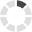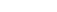### Site InformationLoading... Please wait...
• My Account

# ECON 102 Economics 2 – Microeconomics Quiz 8

\$15.00
Quantity:## Product Description

The law of diminishing returns explains why the long-run average total cost curve is U- shaped.

The law of diminishing returns explains why short-run marginal cost curves are upward sloping.

Minimum efficient scale varies by industry

Which of the following statements concerning the relationships between total product (TP), average product (AP), and marginal product (MP) is not correct?

Answer the next question(s) on the basis of the accompanying table that shows average total costs (ATC) for a manufacturing firm whose total fixed costs are \$10:

Suppose that, when producing 10 units of output, a firm's AVC is \$22, its AFC is \$5, and its MC is \$30. This:

The short run is characterized by: Select one:

In economics, a firm earns a normal profit when its total revenue equals its total economic costs.

In the above diagram it is assumed that: Select one:

The long-run average total cost curve: Select one:

Refer to the above graph. Which one of the following would cause a move from point b to point c along short-run average total cost curve ATC1?

Economic profits are calculated by subtracting: Select one:

A firm's total variable cost will depend on: Select one:

(Last Word) Which of the following sayings relates most closely to the idea of sunk costs: Select one:

Assume a firm closes down in the short run and produces no output. Under these conditions:

Refer to the above diagram, where variable inputs of labor are being added to a constant amount of property resources. The total output of this firm will cease to expand:

Answer the next question(s) on the basis of the following output data for a firm. Assume that the amounts of all non-labor resources are fixed.

Refer to the above graph. Which one of the following would cause a move from point d to point e along short-run average total cost curve ATC2?

Average fixed costs diminish continuously as output increases. Select one:

## Product Reviews

This product hasn't received any reviews yet. Be the first to review this product!

## Customers also viewed

• \$15.00• \$20.00• \$20.00• \$10.00• \$10.00## Related Products

Click the button below to add the ECON 102 Economics 2 – Microeconomics Quiz 8 to your wish list.